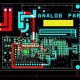# Very Simple Tutorial about How to Convert AC into DC.

In this tutorial, we are going to learn about how to convert AC into DC.

Below is the Main Step for Convert AC into DC.

• Stepping Down the Voltage Level.
• AC to DC Power Converter Circuit.
• Obtaining Pure DC from Pulsating DC.
• Regulating DC voltage.

Stepping Down the Voltage Level:

By using step down transformer, we can step down the voltage like we have power 230 AC voltage then if we have requirement of 12V then we can use step down transformer 230 VAC to 12VAC.

AC to DC Power Converter Circuit:

Converting alternating current into direct current is given the name rectification. For rectification we use A P-N junction diode conduct current only in one direction. The same principal is use of in a rectifier to convert AC to DC. When positive supply comes then P junction become forward bias and N junction become reverse bias, when negative power comes then N junction become forward bias and P junction become reverse bias. Basically we use half wave rectifier and full wave rectifier.

Obtaining Pure DC from Pulsating DC:

When convert AC into DC by rectifier then DC voltage is not getting pure there is some AC voltage so that we use filter for pure DC, In filter when capacitor charges up the voltage from the rectifier rises above the capacitor voltage and then as the rectifier voltage falls, the capacitor provides the required current form its stored charge. Ripple voltage is the residual periodic variation of the DC voltage.

Regulating DC voltage:

Step down voltage as per requirement by using voltage regulator like if we want 12V then we will use 12V regulator and if we want will use 5V regulator like LM7805 is 5V voltage regulator which give the fix output 5V also we can use ZENER for voltage regulation but there is not stability so that we use mostly regulator.

Application of AC to DC converter:

• All types of Led Driver.
• All types of Mobile Charger.
• All types of laptop charger.
• All types of consumer item which are operated at Voltage.